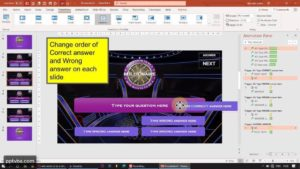# INTERACTIVE POWERPOINT QUIZ GAME REPORT CARD

This PowerPoint Quiz Game is going to calculate the Number of Questions answered correctly, wrongly, and passed. The VBA code also calculates the Points and the Percentage that had been secured by the student who attempted the PPT Quiz Game.

In the module, we will be adding more ActiveX Labels in the Slide Master that we made in Part 1 where only the Points were calculated. We are going to have exclusive labels for:

• Passed Questions
• Total Questions
• Points
• Percentage

We also add the feature to pass a question. The student needn’t guess and attempt the question by guessing and save the potential negative marking.

In the second video, I show how we can make an Interactive PowerPoint Quiz Game that could calculate and generate the Grade in the PowerPoint Quiz Report Card using VBA. Hint: We use If-Then Conditions based on the percentage.

Also, I received lot of feedback that the default Message Box (MsgBox) present in PowerPoint VBA that I use currently was very bland and boring.

I decided to fix that by having the answer shape change colours when they are clicked. So, Instead of using a MsgBox to indicate whether the answer is correct, we use Trigger Emphasis Animations.

Due to that, I was also able to add the feature of having multiple attempts to answer the question. At the same time, only the first attempt is calculated for the report-card. This would allow the student to know the correct answer while playing the PowerPoint Quiz Game.

Send Report Card to Google Sheets, Import Questions from Excel.
Make your quiz game in 54 seconds!

## Adding a Report Card in Microsoft PowerPoint Quiz using VBA

We create a slide-master layout within which we place multiple Labels along with the Points Label that can keep count of number of questions that have been answered correctly and the number of questions that have been answered incorrectly.

Similarly, we add more ActiveX Labels for Passed Questions, Total Questions, Percentage, Points and Grade*.

There is no reason to technically use a Slide Master. You can add the labels in a normal slide too. The only reason I made it in a Slide Master was to just keep it organised and have all my codes within a SlideLayout and not in separate slides.

## Multiple Attempts to answer Question in PowerPoint Quiz Game

Since I did not automatically take the student to the next question slide, they will have the ability to click on another answer again.

In my premium template, I overcame this by adding a transparent shape over all the questions which is hidden by default but is visible when any of the answer is pressed. I use the .Shapes.Visible = msoTrue / msoFalse in VBA. Also, this is customisable, the teacher can choose to enable it.

In my tutorial video however, I allowed the students to attempt the question multiple times. Only the first response would be taken into consideration for the report card. This was possible using a Boolean and If-Then Conditions based on the value of the Boolean.

To calculate the Grade in PowerPoint, we first need to calculate the percentage:
``````Sub Percentage()
C = Int(CA.Caption)
W = Int(WA.Caption)
TQ = C + W
P.Caption = Round(C / TQ * 100, 1) 'rounds 1st decimal'
End Sub ``````

We use the Round() function to round-off our percentage. Currently, it rounds off to the first decimal.

Now that we have calculated our Percentage by dividing the number of correct answers by the total number of questions and multiplying by 100, we can now determine the grade in this PowerPoint Quiz Game using If-Then Conditions:

``````Sub Grade()
'P.Caption refers to the Percentage ActiveX Label'
'G.Caption refers to the Grade ActiveX Label'
If P.Caption >= 0 Then G.Caption = "F"
If P.Caption > 40 Then G.Caption = "E"
If P.Caption > 50 Then G.Caption = "D"
If P.Caption > 70 Then G.Caption = "C"
If P.Caption > 80 Then G.Caption = "B"
If P.Caption > 90 Then G.Caption = "A"
End Sub ``````

### Powerpoint vba code for quiz game

``````'without grade'

Sub Correct()
Points.Caption = (Points.Caption) + 10
CA.Caption = (CA.Caption) + 1
ActivePresentation.SlideShowWindow.View.Next
End Sub

Sub Wrong()
Points.Caption = (Points.Caption) - 5
WA.Caption = (WA.Caption) + 1
ActivePresentation.SlideShowWindow.View.Next
End Sub

Sub ResetAllCaptions()
CA.Caption = 0
WA.Caption = 0
PQ.Caption = 0
TQ.Caption = 0
Percentage.Caption = 0
Points.Caption = 0
End Sub

Sub Reset()
ResetAllCaptions
ActivePresentation.SlideShowWindow.View.Exit
End Sub

Sub Retry()
ResetAllCaptions
ActivePresentation.SlideShowWindow.View.GotoSlide (1)
End Sub

Sub ShowResult()
TQ.Caption = 6 'change accordingly as per your pptm file'
PQ.Caption = Int((TQ.Caption) - (CA.Caption) - (WA.Caption))
Percentage.Caption = (CA.Caption) * 100 / (TQ.Caption)
ActivePresentation.SlideShowWindow.View.Next
End Sub ``````
``````'with grade'

Dim QA As Boolean

If QA = False Then CA.Caption = (CA.Caption) + 1
QA = True
End Sub

If QA = False Then WA.Caption = (WA.Caption) + 1
QA = True
End Sub

Sub Percentage()
C = Int(CA.Caption)
W = Int(WA.Caption)
TQ = C + W
P.Caption = Round(C / TQ * 100, 1)
End Sub

Sub NextQuestion()
QA = False
ActivePresentation.SlideShowWindow.View.Next
End Sub

If P.Caption >= 0 Then G.Caption = "F"
If P.Caption > 40 Then G.Caption = "E"
If P.Caption > 50 Then G.Caption = "D"
If P.Caption > 70 Then G.Caption = "C"
If P.Caption > 80 Then G.Caption = "B"
If P.Caption > 90 Then G.Caption = "A"
End Sub

Sub CalculateResult()
Percentage
ActivePresentation.SlideShowWindow.View.Next
End Sub

Sub Initialise()
CA.Caption = 0
WA.Caption = 0
P.Caption = 0
G.Caption = 0
End Sub

Sub EndGame()
Initialise
ActivePresentation.SlideShowWindow.View.Exit
End Sub

Sub StartGame()
QA = False
Initialise
ActivePresentation.SlideShowWindow.View.Next
End Sub
``````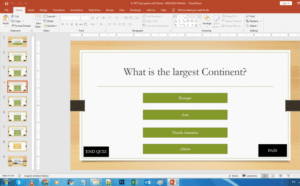## Interactive PowerPoint Quiz Game with Points and Scores

In this PowerPoint Quiz Module, we will be designing our...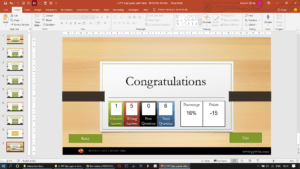## REPORT CARD AND PERCENTAGE – INTERACTIVE POWERPOINT QUIZ GAME

In this PowerPoint Quiz Game, we will calculate the number...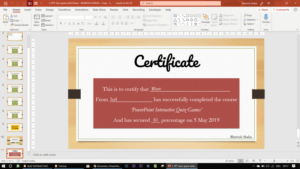## PRINT CERTIFICATE IN PPT QUIZ GAME

Collect student's name via an input field and use VBA...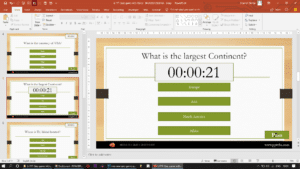## POWERPOINT QUIZ GAME WITH TIMER

As soon as the time limit is up, the student...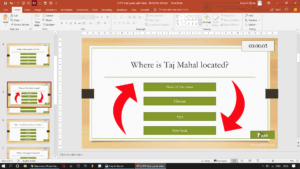## SHUFFLE QUESTIONS SLIDES & ANSWER ORDER RANDOMLY IN PPT QUIZ GAME​

We will be shuffling the order of the questions such...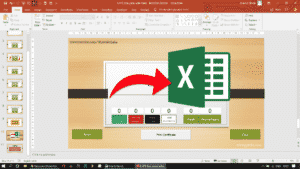## SAVE POWERPOINT QUIZ RESULTS TO EXCEL SHEET​

We will be exporting/sending the results of the PowerPoint Quiz...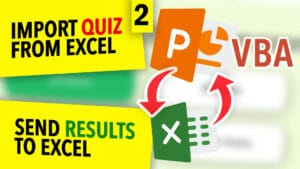## Populate A PowerPoint Quiz From Excel With VBA

Import Questions & Answers of a Microsoft PowerPoint Multiple-Choice Quiz...